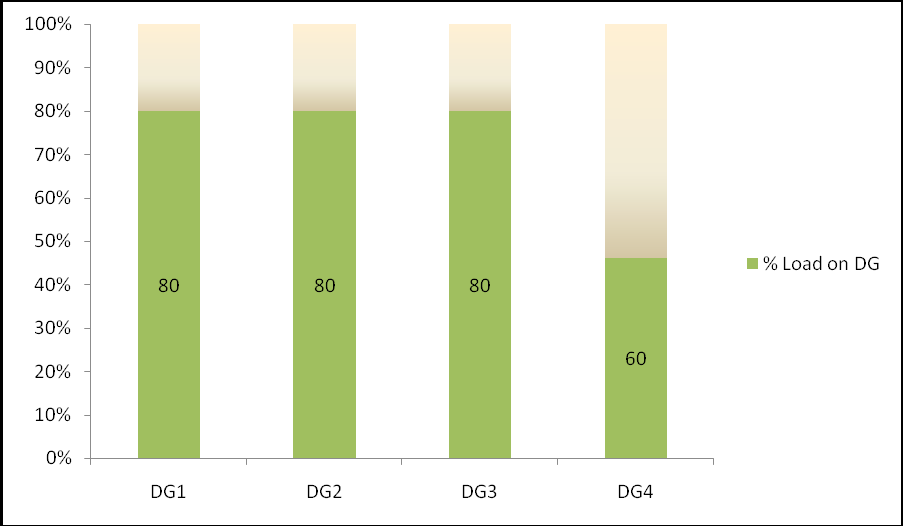When asymmetric LS is enabled in menu 8280, the ‘normal’ G5 load sharing is deactivated in
all AGC units in the system. The AGC units will then load share according to the asymmetric
LS set point in menu 8280.
Example: If 4 no of DG’s each having 1000KW nominal power. Asymmetric load set point is
80% and load on the bus bar is 3000 KW.Then Load sharing will be as followsAs shown above DG1, DG2 and DG3 will take 80 %( i.e. 800KW) load and last priority DG4 will take remaining 60% load (i.e. 600KW).

Hence DG1 = 800 KW
DG2 = 800 KW
DG3 = 800 KW
DG4 = 600 KW